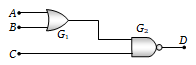• Subject:

...

• Topic:

...

For the given combination of gates, if the logic states of inputs A, B, C are as follows A = B = C = 0 and A = B = 1, C = 0 then the logic states of output D are(a) 0, 0
(b) 0, 1
(c) 1, 0
(d) 1, 1